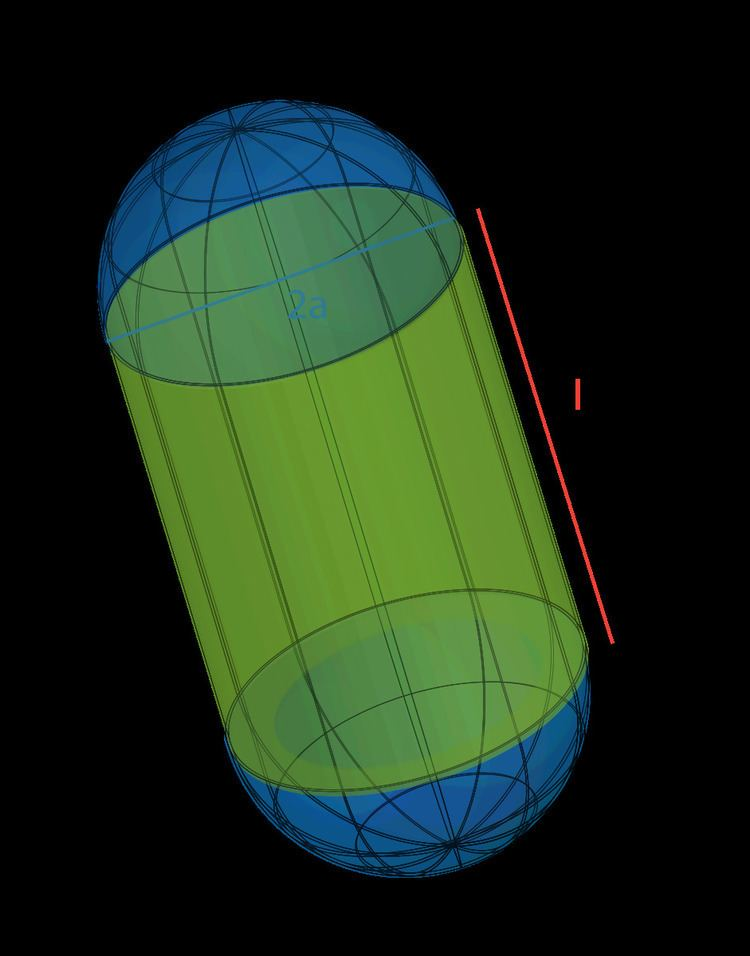# Hille equation

Updated onThe Hille equation relates the maximum ionic conductance of an ion channel to its length and radius (or diameter), with the commonly used version implicitly takes into account a hemispherical cap. As it is ultimately based on a macroscopic continuum model, it does not take into account molecular interactions, and real conductances are often several times less than the predicted maximal flux.

## Equation

The Hille equation predicts the following maximum conductance g for a pore with length l , radius a , in a solvent with resistivity ρ :

1 g = ( l + π a 2 ) × ρ π a 2

Rearranging the terms, the maximal flux based on length l and diameter d can be shown to be:

1 g = l ρ ( π ( d 2 ) 2 ) + ρ d

## References

Similar Topics
Snowbound (1948 film)
Mike Morgan (politician)
Boris Kitka
Topics